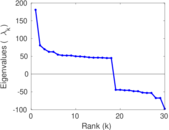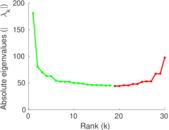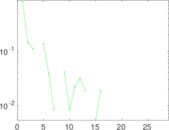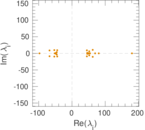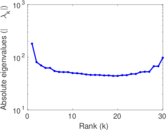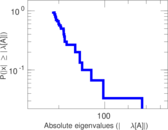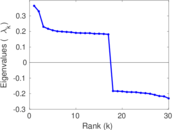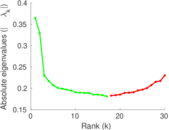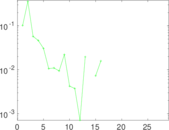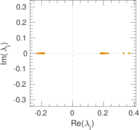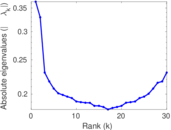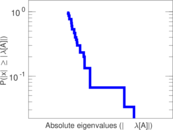# Libimseti.cz

Libimseti.cz (also spelled Líbímseti.cz; from Czech Líbím se ti, "Do you like me") is a Czech dating site. This is the network of ratings given by users of Libimseti.cz to other users. The network is unipartite, directed, and edges represent ratings on a scale from 1 to 10.

 Code `LI` Internal name `libimseti` Name Libimseti.cz Data source http://www.occamslab.com/petricek/data/ AvailabilityDataset is available for download Consistency checkDataset passed all tests Category Online social network Node meaning User Edge meaning Rating Network format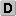Unipartite, directed Edge type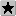Ratings, no multiple edges ReciprocalContains reciprocal edges Directed cyclesContains directed cycles LoopsDoes not contain loops

## Statistics

 Size n = 220,970 Volume m = 17,359,346 Loop count l = 0 Wedge count s = 28,261,600,655 Claw count z = 82,085,727,142,249 Cross count x = 328,616,649,550,852,928 Triangle count t = 69,115,603 Square count q = 252,125,304,066 4-Tour count T4 = 2,130,083,301,436 Maximum degree dmax = 33,389 Maximum outdegree d+max = 25,042 Maximum indegree d−max = 33,389 Average degree d = 157.119 Fill p = 0.000 355 524 Size of LCC N = 220,970 Size of LSCC Ns = 81,145 Relative size of LSCC Nrs = 0.367 222 Diameter δ = 6 50-Percentile effective diameter δ0.5 = 2.383 02 90-Percentile effective diameter δ0.9 = 3.088 97 Median distance δM = 3 Mean distance δm = 2.853 61 Gini coefficient G = 0.710 031 Balanced inequality ratio P = 0.232 265 Outdegree balanced inequality ratio P+ = 0.288 021 Indegree balanced inequality ratio P− = 0.176 887 Relative edge distribution entropy Her = 0.905 698 Power law exponent γ = 1.267 38 Tail power law exponent γt = 1.911 00 Degree assortativity ρ = −0.138 967 Degree assortativity p-value pρ = 0.000 00 In/outdegree correlation ρ± = −0.053 936 3 Clustering coefficient c = 0.007 336 70 Directed clustering coefficient c± = 0.029 130 8 Spectral norm α = 1,127.41 Operator 2-norm ν = 1,091.14 Cyclic eigenvalue π = 180.755 Spectral separation |λ1[A] / λ2[A]| = 1.048 45 Reciprocity y = 0.014 539 9 Non-bipartivity bA = 0.167 400 Normalized non-bipartivity bN = 0.133 489 Negativity ζ = 0.478 986 Algebraic conflict ξ = 0.335 197 Triadic conflict τ = 0.523 841 Spectral signed frustration φ = 0.000 537 773

## Plots

### Degree distribution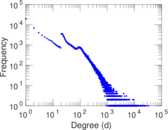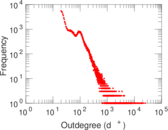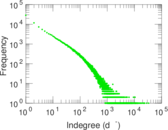### Cumulative degree distribution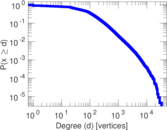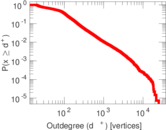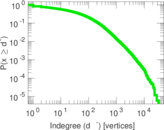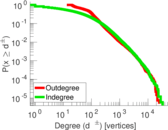### Lorenz curve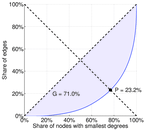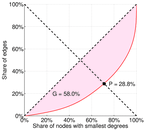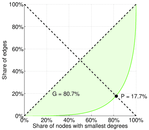### Spectral distribution of the adjacency matrix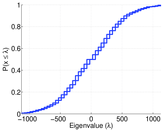### Spectral distribution of the normalized adjacency matrix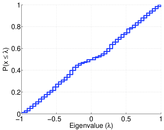### Spectral distribution of the Laplacian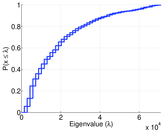### Spectral graph drawing based on the adjacency matrix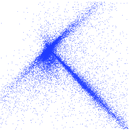### Spectral graph drawing based on the normalized adjacency matrix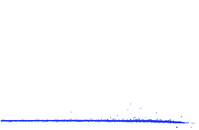### Zipf plot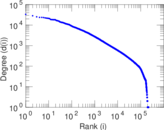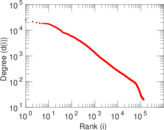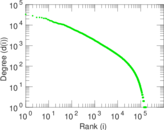### Hop distribution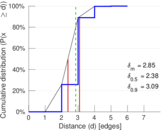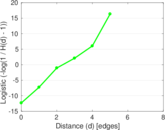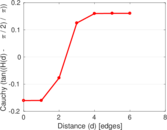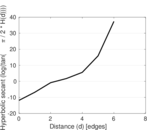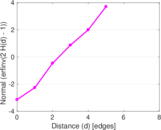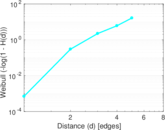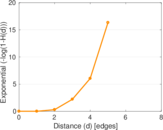### In/outdegree scatter plot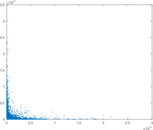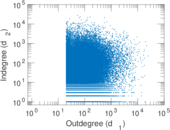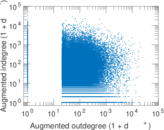### Item rating evolution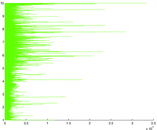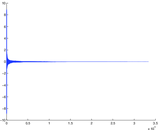### Edge weight/multiplicity distribution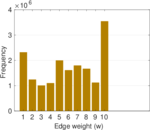### Clustering coefficient distribution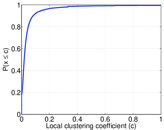### SynGraphy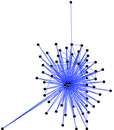### Matrix decompositions plots